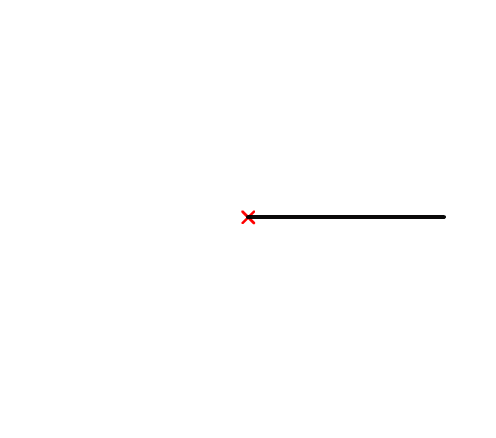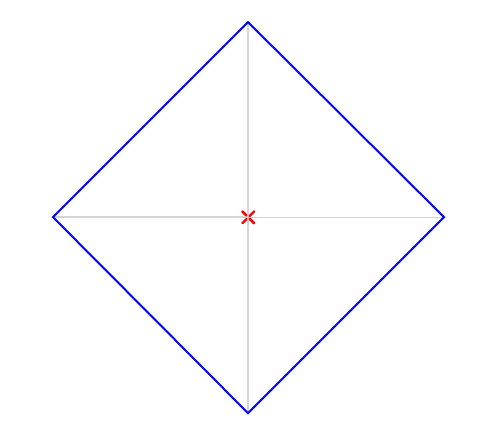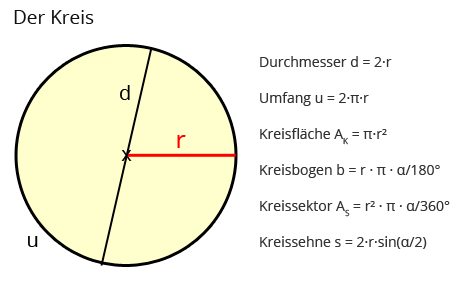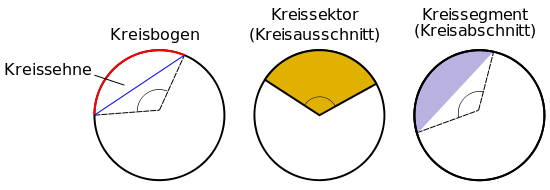# What is the meaning of circles

## Circle - definition and characteristics

The circle is a flat geometric figure with an infinite number of points at the same distance from the center point and resulting in a circular line.

The area enclosed by the circular line is called the circular area. The distance to the center is called the radius.If we were to extend the definition to a space, we would speak of a sphere.

If one already knows limit values, one can also define that the circle is a regular polygon that consists of an infinite number of sides. The following animation shows this:### Features of the circle

• The circle has no corners, an infinite number of sides (the circular line) and 1 area.
• The circle has no interior angles.
• The circle is point-symmetrical to its origin.
• All lines from one point through the center point to the opposite point are of the same length (the diameters).
• A line from the center point to another point on the circle is called a radius. It is half the length of the diameter.
• A circle does not belong to the group of polygons (polygons).

### Circle illustration with formulas